output.to from Sideway
Draft for Information Only

# Content

``` Centroid of Volume by Geometric Decomposition   Centroids of Composite 3D Body   Centroid of composite 3D body 1   Centroid of composite 3D body 2```

# Centroid of Volume by Geometric Decomposition

By denoting the position of the element of a 3D body relative to the coordinate system as xi,yi,zk, the coordinates x, y, z , of the center of gravity of the plane body where the resultant force W applied can be determined by equating the moment of the resultant force W about the yz plane, zx plane and xy plane and the corresponding mements of elements of the 3D body about the coordinate axis respectively. Imply.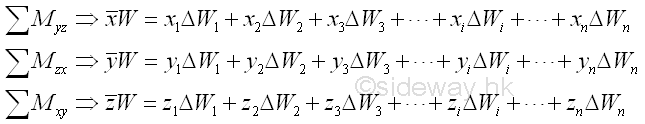Therefore the coordinates x , y , and  z of the center of gravity of the plane body is.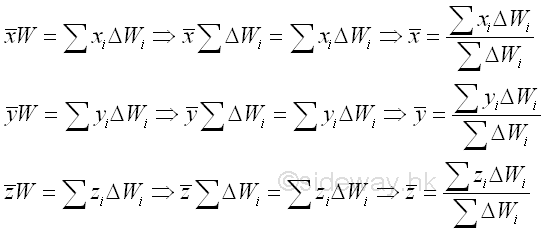If a 3D body can be divided into some common 3D bodys, the 3D body can be considered as a composite 3D body. For a composite 3D body, the cooresponding mements of elements of the plane body can also be grouped according to the composition of the 3D body. Imply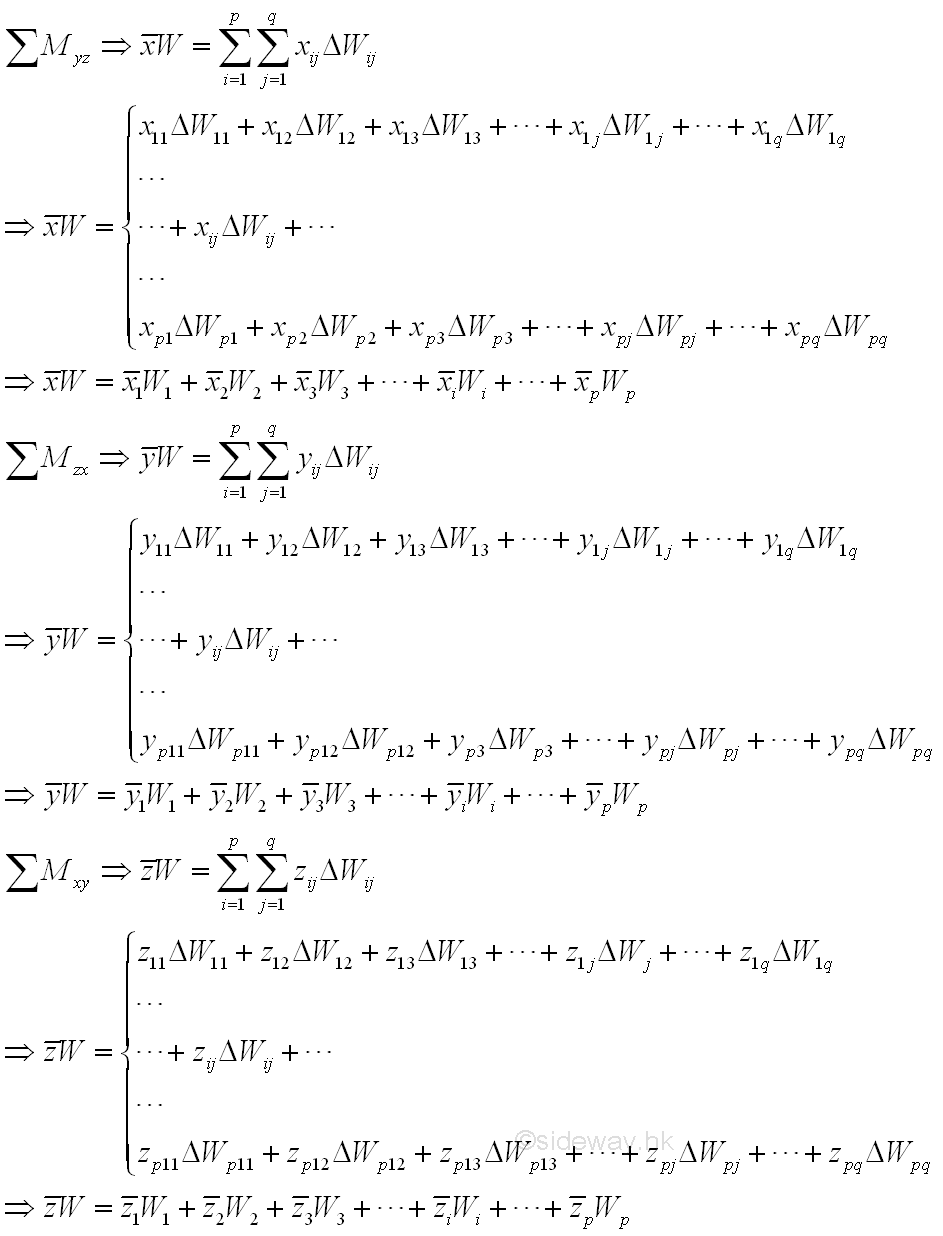For a homogeneous 3D body, the first moment of the 3D body volume is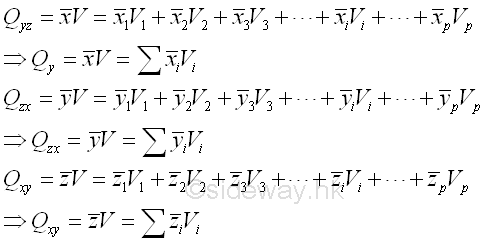## Centroids of Composite 3D Body

### Centroid of composite 3D body 1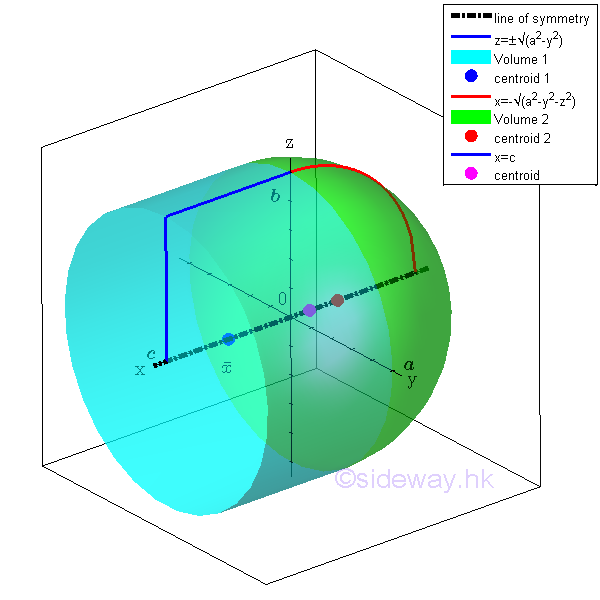For example, the signed volume of a composite 3D body bounded by surfaces in rectangular form , Imply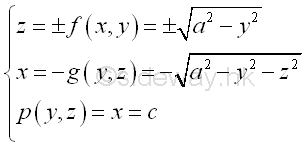The composite 3D volume can be divided into two common shapes. Therefore, centroid of the bounded volume by concept of composite 3D body is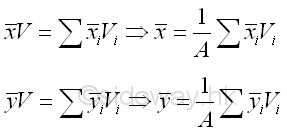The volume of the 3D body is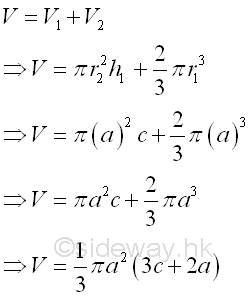Coordinate x of centroid

The first moment of area can be either positive and negative. The centroid of volume 1 is located to the positive side of the yz plane, the first moment of volume is positive.  Since the centroid of volume 2 is located to the negative side of the yz plane, the first moment of area is negative and the negative sign is usually assigned to the x coordinate of the centroid of volume 2. Imply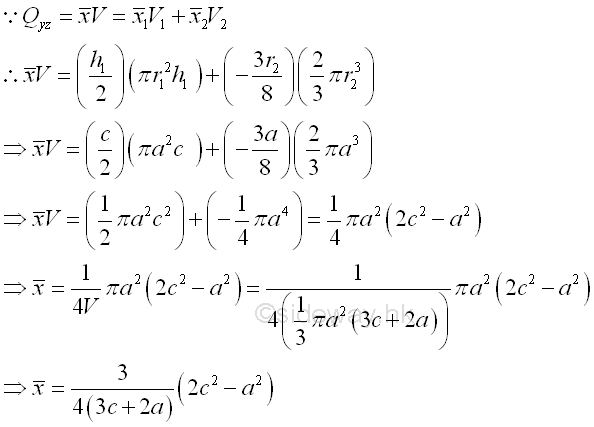Similarly if the coordinate x of centroid of 3D volume is positive, the coordinate x of the centroid is located to the positive side of yz plane.

Coordinate y and z of  centroid

By symmetry, the coordinate y and  z of centroid lie on the x axis, the line of symmetry.

### Centroid of composite 3D body 2

Since the first moment of volume of a hole can be considered as the negative sense of the first moment of volume by assuming the first moment of volume of the same volume of negative sense. Therefore the first moment of volume of a hole is negative. The negative sign is usually assigned to the volume of the hole and the volume of a hole is called negative volume.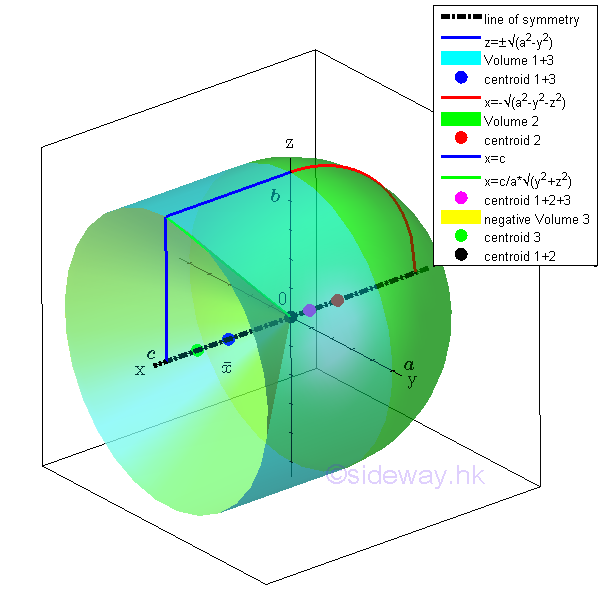For example, the signed volume of a composite 3D volume bounded by surfaces in rectangular form , Imply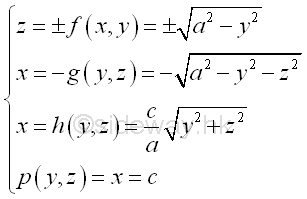The 3D volume body can be divided into common shapes. Therefore, centroid of the bounded voulme by concept of composite 3D volume body isThe volume of 3D body is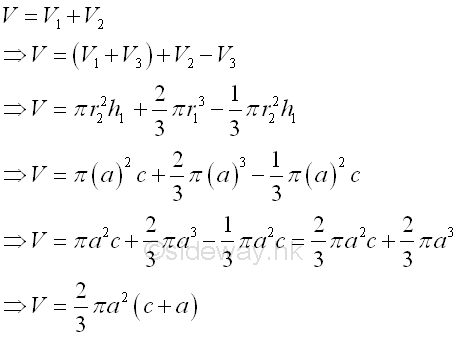Coordinate x of centroid

The first moment of volume can be either positive and negative. The centroid of volume 1 plus volume 3 is located to the positive side of the yz planes, the first moment of volume is positive.  Since the centroid of volume 2 is located to the negative of the yz planes, the first moment of volume is negative and the negative sign is usually assigned to the x coordinate of the centroid of volume 1. And for the centroid of hole, negative volume 3 is located to the positive side of the yz planes, the coordinate of the centroid of volume 3 is positive but the sense of the volume is negative. ImplyIf c is equal to a, the coordinate x of centroid of volume of the 3D body is located on the origin 0 of x axis.

Coordinate y and z of  centroid

By symmetry, the coordinate y and  z of centroid lie on the x axis, the line of symmetry.

ID: 120700004 Last Updated: 12/7/2012 Revision: 0 Ref:References

1. F.P. Beer; E.R. Johnston,Jr.; E.R. Eisenberg, 2004, Vector Mechanics for Engineers: Statics
2. I.C. Jong; B.G. rogers, 1991, Engineering Mechanics: Statics and DynamicsHome 5

Management

HBR 3

Information

Recreation

Culture

Chinese 1097

English 337

Computer

Hardware 151

Software

Application 202

Latex 10

Manim 159

Numeric 19

Programming

Web 285

Unicode 494

HTML 65

CSS 59

ASP.NET 194

OS 391

Python 36

Knowledge

Mathematics

Algebra 29

Geometry 18

Calculus 67

Engineering

Mechanical

Rigid Bodies

Statics 92

Dynamics 37

Control

Natural Sciences

Electric 27